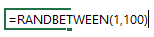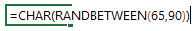This post is about the RAND and the RANDBETWEEN functions of Excel and how can we play with these to

• Generate random Alphabets (+ its variations) or
• Generate random numbers (+ variations)
• Generate random names from a ranges
• Generate random dates

There will be a lot more things that you can generate randomly after you read this post. Let’s get going!

## #1 How to Generate Random Numbers

Not only I am going to show you how to generate random numbers but also a few variations that you can choose from.

Use this formula to generate random numbers between 1 – 100Note a few things

1. Just like this you can generate random numbers between any two numbers (positive or negative)
2. The random numbers will keep on refreshing every time the sheet gets refreshed
3. If you want static numbers, copy the numbers and paste them as values using paste special

You can also use the RAND Function to generate random numbers between 1 and 100Use this formula to generate random roman numbers between 1 – 100## #2 How to Generate Random Alphabets (Letters)

Use this formula to generate alphabets (between A – Z) upper caseA little tweak in this formula can generate random alphabets between (a – z) lower caseNotes

1. If you are wondering what are the numbers 65 and 90. They are the ASCII codes for capital A and capital Z
2. Similarly 97 and 122 are the ASCII codes for small a and small z
3. The CHAR function coverts the number to a character

## #3 Generate Random Names / Items from a Range

This formula will return a random name from the rangeNote that :

1. The range has been named as Names
2. Using the COUNTA function to count the number of names (text) in the range
3. Then using the RANDBETWEEN Function to pull out a random number
4. Then applying the INDEX Function to pull out the name

## #4 Generate Random Dates

Let’s assume that we want to generate any random date in the year 2016Note that

1. The DATE function generates a date
2. When you confirm the formula, it will result in a number
3. Don’t forget to convert that number in a date format (Shortcut : Ctrl + Shift + 3)

## #5 Generate Random Cell Addresses

Let’s assume that we want to get a random cell address between A1:A100. Here goes the formulaI have used the combination of RANDBETWEEN and the ADDRESS function here

## Ever used any of these?

Let me know if you find a good use of any of these in your work. Are there any more rand function tricks that you use? Please put them down in the comments